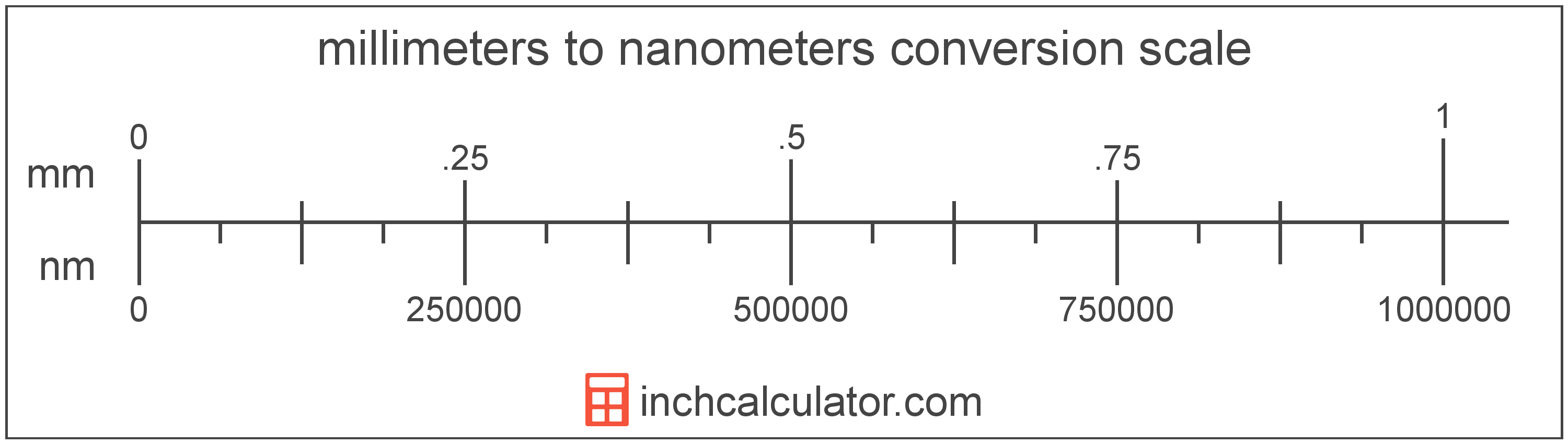# Nanometers to Millimeters Converter

Enter the length in nanometers below to get the value converted to millimeters.

Results in Millimeters:1 nm = 1.0E-6 mm
Hint: use a scientific notation calculator to convert E notation to decimal

Do you want to convert millimeters to nanometers?

## How to Convert Nanometers to Millimeters

To convert a measurement in nanometers to a measurement in millimeters, divide the length by the following conversion ratio: 1,000,000 nanometers/millimeter.

Since one millimeter is equal to 1,000,000 nanometers, you can use this simple formula to convert:

millimeters = nanometers ÷ 1,000,000

The length in millimeters is equal to the length in nanometers divided by 1,000,000.

For example, here's how to convert 5,000,000 nanometers to millimeters using the formula above.
millimeters = (5,000,000 nm ÷ 1,000,000) = 5 mmOur inch fraction calculator can add nanometers and millimeters together, and it also automatically converts the results to US customary, imperial, and SI metric values.

## What is a Nanometer?

One nanometer is equal to one-billionth (1/1,000,000,000) of a meter, which is defined as the distance light travels in a vacuum in a 1/299,792,458 second time interval.

The nanometer, or nanometre, is a multiple of the meter, which is the SI base unit for length. In the metric system, "nano" is the prefix for billionths, or 10-9. Nanometers can be abbreviated as nm; for example, 1 nanometer can be written as 1 nm.

The nanometer is an extremely small unit of length measurement, often used to measure things that are very small, such as the transistors and electrical pathways in computer processors and nanotechnology.

## What is a Millimeter?

One millimeter is equal to one-thousandth (1/1,000) of a meter, which is defined as the distance light travels in a vacuum in 1/299,792,458 of a second. One millimeter is equal to 0.0393701 inches.

The millimeter, or millimetre, is a multiple of the meter, which is the SI base unit for length. In the metric system, "milli" is the prefix for thousandths, or 10-3. Millimeters can be abbreviated as mm; for example, 1 millimeter can be written as 1 mm.

Millimeters are often represented by the smallest ticks on most metric rulers. To get a reference point of the size, the thickness of a US dime is 1.35mm.

We recommend using a ruler or tape measure for measuring length, which can be found at a local retailer or home center. Rulers are available in imperial, metric, or a combination of both values, so make sure you get the correct type for your needs.

Need a ruler? Try our free downloadable and printable rulers, which include both imperial and metric measurements.

## Nanometer to Millimeter Conversion Table

Table showing various nanometer measurements converted to millimeters.
Nanometers Millimeters
1 nm 0.000001 mm
2 nm 0.000002 mm
3 nm 0.000003 mm
4 nm 0.000004 mm
5 nm 0.000005 mm
6 nm 0.000006 mm
7 nm 0.000007 mm
8 nm 0.000008 mm
9 nm 0.000009 mm
10 nm 0.00001 mm
100 nm 0.0001 mm
1,000 nm 0.001 mm
10,000 nm 0.01 mm
100,000 nm 0.1 mm
1,000,000 nm 1 mm

## References

1. United States Mint, Coin Specifications, https://www.usmint.gov/learn/coin-and-medal-programs/coin-specifications